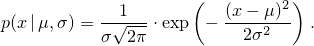# 26 Numerics library [numerics]

## 26.5 Random number generation [rand]

### 26.5.8 Random number distribution class templates [rand.dist]

#### 26.5.8.5.1 Class template normal_distribution[rand.dist.norm.normal]

A normal_distribution random number distribution produces random numbers x distributed according to the probability density functionThe distribution parameters μ and σ are also known as this distribution's mean and standard deviation.

template<class RealType = double>
class normal_distribution{
public:
// types
typedef RealType result_type;
typedef unspecified param_type;

// constructors and reset functions
explicit normal_distribution(RealType mean = 0.0, RealType stddev = 1.0);
explicit normal_distribution(const param_type& parm);
void reset();

// generating functions
template<class URNG>
result_type operator()(URNG& g);
template<class URNG>
result_type operator()(URNG& g, const param_type& parm);

// property functions
RealType mean() const;
RealType stddev() const;
param_type param() const;
void param(const param_type& parm);
result_type min() const;
result_type max() const;
};


 explicit normal_distribution(RealType mean = 0.0, RealType stddev = 1.0); 

Requires: 0 < stddev .

Effects: Constructs a normal_distribution object; mean and stddev correspond to the respective parameters of the distribution.

 RealType mean() const; 

Returns: The value of the mean parameter with which the object was constructed.

 RealType stddev() const; 

Returns: The value of the stddev parameter with which the object was constructed.